/

### The t copula

The t copula is the copula that underlies the multivariate Student’s t distribution.

 Copula name t copula Common notation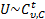Parameters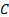, a non-negative definite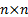matrix, i.e. a matrix that can correspond to a correlation matrix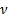= degrees of freedom (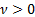, usuallyis an integer although in some situations a non-integralcan arise) (note in principle each marginal distribution could in principle have a different number of degrees of freedom although such a refinement is not commonly seen) Domain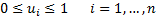Copula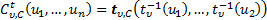where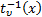is the inverse student’s t function and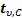is the cumulative distribution function of the multivariate student’s t distribution with arbitrary mean and matrix generator equal toKendall’s rank correlation coefficient (for bivariate case),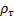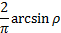Where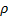is the correlation coefficient between the two variables Coefficient of upper tail dependence,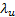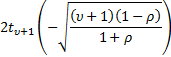Coefficient of lower tail dependence,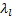Other comments If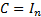(theidentity matrix) then, in contrast to the Gaussian copula, we do not recover the independence copula.

Nematrian web functions

Functions relating to the above distribution in the two-dimensional case may be accessed via the Nematrian web function library by using a DistributionName of “student’s t (2d)”. For details of other supported probability distributions see here.